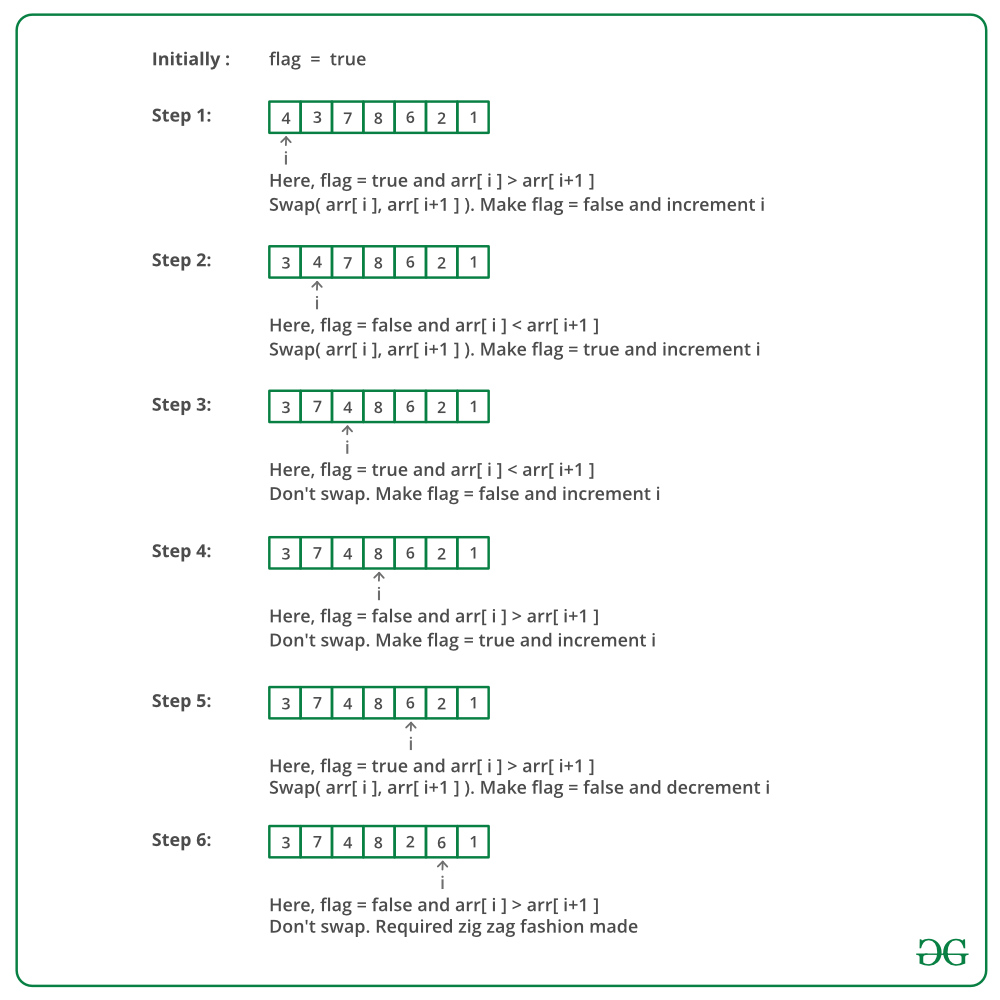Related Articles

# Convert array into Zig-Zag fashion

• Difficulty Level : Easy
• Last Updated : 18 May, 2021

Given an array of DISTINCT elements, rearrange the elements of array in zig-zag fashion in O(n) time. The converted array should be in form a < b > c < d > e < f

Example:

Input: arr[] = {4, 3, 7, 8, 6, 2, 1}
Output: arr[] = {3, 7, 4, 8, 2, 6, 1}

Input: arr[] = {1, 4, 3, 2}
Output: arr[] = {1, 4, 2, 3}

A Simple Solution is to first sort the array. After sorting, exclude the first element, swap the remaining elements in pairs. (i.e. keep arr as it is, swap arr and arr, swap arr and arr, and so on).
Time complexity: O(N log N) since we need to sort the array first.

We can convert in O(n) time using an efficient approach. The idea is to use a modified one pass of bubble sort.

• Maintain a flag for representing which order(i.e. < or >) currently we need.
• If the current two elements are not in that order then swap those elements otherwise not.

Let us see the main logic using three consecutive elements A, B, C.

Suppose we are processing B and C currently and the current relation is ‘<‘, but we have B > C. Since current relation is ‘<‘ previous relation must be ‘>’ i.e., A must be greater than B. So, the relation is A > B and B > C. We can deduce A > C. So if we swap B and C then the relation is A > C and C < B. Finally we get the desired order A C B
Refer this for more explanation.

Below image is a dry run of the above approach:Below is the implementation of above approach:

## C++

 `// C++ program to sort an array in Zig-Zag form``#include ``using` `namespace` `std;` `// Program for zig-zag conversion of array``void` `zigZag(``int` `arr[], ``int` `n)``{``    ``// Flag true indicates relation "<" is expected,``    ``// else ">" is expected. The first expected relation``    ``// is "<"``    ``bool` `flag = ``true``;` `    ``for` `(``int` `i=0; i<=n-2; i++)``    ``{``        ``if` `(flag) ``/* "<" relation expected */``        ``{``            ``/* If we have a situation like A > B > C,``            ``we get A > B < C by swapping B and C */``            ``if` `(arr[i] > arr[i+1])``                ``swap(arr[i], arr[i+1]);``        ``}``        ``else` `/* ">" relation expected */``        ``{``            ``/* If we have a situation like A < B < C,``            ``we get A < C > B by swapping B and C */``            ``if` `(arr[i] < arr[i+1])``                ``swap(arr[i], arr[i+1]);``        ``}``        ``flag = !flag; ``/* flip flag */``    ``}``}` `// Driver program``int` `main()``{``    ``int` `arr[] = {4, 3, 7, 8, 6, 2, 1};``    ``int` `n = ``sizeof``(arr)/``sizeof``(arr);``    ``zigZag(arr, n);``    ``for` `(``int` `i=0; i

## Java

 `// Java program to sort an array in Zig-Zag form``import` `java.util.Arrays;` `class` `Test``{``    ``static` `int` `arr[] = ``new` `int``[]{``4``, ``3``, ``7``, ``8``, ``6``, ``2``, ``1``};``    ` `    ``// Method for zig-zag conversion of array``    ``static` `void` `zigZag()``    ``{``        ``// Flag true indicates relation "<" is expected,``        ``// else ">" is expected. The first expected relation``        ``// is "<"``        ``boolean` `flag = ``true``;``        ` `        ``int` `temp =``0``;``    ` `        ``for` `(``int` `i=``0``; i<=arr.length-``2``; i++)``        ``{``            ``if` `(flag) ``/* "<" relation expected */``            ``{``                ``/* If we have a situation like A > B > C,``                ``we get A > B < C by swapping B and C */``                ``if` `(arr[i] > arr[i+``1``])``                ``{``                    ``// swap``                    ``temp = arr[i];``                    ``arr[i] = arr[i+``1``];``                    ``arr[i+``1``] = temp;``                ``}``                ` `            ``}``            ``else` `/* ">" relation expected */``            ``{``                ``/* If we have a situation like A < B < C,``                ``we get A < C > B by swapping B and C */``                ``if` `(arr[i] < arr[i+``1``])``                ``{``                    ``// swap``                    ``temp = arr[i];``                    ``arr[i] = arr[i+``1``];``                    ``arr[i+``1``] = temp;``                ``}``            ``}``            ``flag = !flag; ``/* flip flag */``        ``}``    ``}``    ` `    ``// Driver method to test the above function``    ``public` `static` `void` `main(String[] args)``    ``{``        ``zigZag();``        ``System.out.println(Arrays.toString(arr));``    ``}``}`

## Python

 `# Python program to sort an array in Zig-Zag form` `# Program for zig-zag conversion of array``def` `zigZag(arr, n):``    ``# Flag true indicates relation "<" is expected,``    ``# else ">" is expected. The first expected relation``    ``# is "<"``    ``flag ``=` `True``    ``for` `i ``in` `range``(n``-``1``):``        ``# "<" relation expected``        ``if` `flag ``is` `True``:``            ``# If we have a situation like A > B > C,``            ``# we get A > B < C``            ``# by swapping B and C``            ``if` `arr[i] > arr[i``+``1``]:``                ``arr[i],arr[i``+``1``] ``=` `arr[i``+``1``],arr[i]``            ``# ">" relation expected``        ``else``:``            ``# If we have a situation like A < B < C,``            ``# we get A < C > B``            ``# by swapping B and C    ``            ``if` `arr[i] < arr[i``+``1``]:``                ``arr[i],arr[i``+``1``] ``=` `arr[i``+``1``],arr[i]``        ``flag ``=` `bool``(``1` `-` `flag)``    ``print``(arr)` `# Driver program``arr ``=` `[``4``, ``3``, ``7``, ``8``, ``6``, ``2``, ``1``]``n ``=` `len``(arr)``zigZag(arr, n)` `# This code is contributed by Pratik Chhajer`

## C#

 `// C# program to sort an array in Zig-Zag form``using` `System;` `class` `GFG{``    ` `static` `int` `[]arr = ``new` `int``[]{ 4, 3, 7, 8, 6, 2, 1 };` `// Method for zig-zag conversion of array``static` `void` `zigZag()``{``    ` `    ``// Flag true indicates relation "<"``    ``// is expected, else ">" is expected.``    ``// The first expected relation``    ``// is "<"``    ``bool` `flag = ``true``;``    ` `    ``int` `temp = 0;` `    ``for``(``int` `i = 0; i <= arr.Length - 2; i++)``    ``{``        ` `        ``// "<" relation expected``        ``if` `(flag)``        ``{``            ` `            ``// If we have a situation like A > B > C,``            ``// we get A > B < C by swapping B and C``            ``if` `(arr[i] > arr[i+1])``            ``{``                ` `                ``// Swap``                ``temp = arr[i];``                ``arr[i] = arr[i + 1];``                ``arr[i + 1] = temp;``            ``}``        ``}``        ` `        ``// ">" relation expected``        ``else``        ``{``            ` `            ``// If we have a situation like A < B < C,``            ``// we get A < C > B by swapping B and C``            ``if` `(arr[i] < arr[i + 1])``            ``{``                ` `                ``// Swap``                ``temp = arr[i];``                ``arr[i] = arr[i + 1];``                ``arr[i + 1] = temp;``            ``}``        ``}``        ` `        ``// Flip flag``        ``flag = !flag;``    ``}``}` `// Driver code``public` `static` `void` `Main(String[] args)``{``    ``zigZag();``    ``foreach``(``int` `i ``in` `arr)``        ``Console.Write(i + ``" "``);``}``}` `// This code is contributed by amal kumar choubey`

## Javascript

 ``

Output:

`3  7  4  8  2  6  1 `

Time complexity: O(n)
Auxiliary Space: O(1)